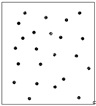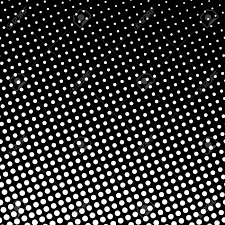# White and black dot detection using OpenCV | Python

Image processing using Python is one of the hottest topics in today’s world. But image processing is a bit complex and beginners get bored in their first approach. So in this article, we have a very basic image processing python program to count black dots in white surface and white dots in the black surface using OpenCV functions (`cv2.imread, cv2.threshold, cv2.findContours, cv2.contourArea`).

## Count black dots on a white surface –

At first we need to import OpenCV library. All functions regarding image processing reside in this library. In order to store the path of the image we are going to process in a variable path.

 `import` `cv2 `

 `# path ="C:/Users/Personal/Downloads/black dot.jpg" ` `path ``=``"black dot.jpg"`

Input Image –Loading an image in grayscale mode. By grayscale mode, the image is converted to a black & white image composing by shades of gray.

 `gray ``=` `cv2.imread(path, ``0``) `

The function `cv2.threshold `works as, if pixel value is greater than a threshold value, it is assigned one value (may be white), else it is assigned another value (may be black). First argument is the source image, which should be a grayscale image(done previously). Second argument is the threshold value which is used to classify the pixel values. For threshold value, simply pass zero. Then the algorithm finds the optimal threshold value and returns you as the second output, th. If Otsu thresholding is not used, th is same as the threshold value you used.

 `# threshold ` `th, threshed ``=` `cv2.threshold(gray, ``100``, ``255``, ` `       ``cv2.THRESH_BINARY_INV|cv2.THRESH_OTSU) `

Contours can be explained simply as a curve joining all the continuous points (along the boundary), having same color or intensity. The contours are a useful tool for shape analysis and object detection and recognition. Contours give better accuracy for using binary images. There are three arguments in `cv2.findContours()` function, first one is source image, second is contour retrieval mode, third is contour approximation method. It outputs the contours and hierarchy. Contours is a Python list of all the contours in the image. Each individual contour is a Numpy array of (x, y) coordinates of boundary points of the object.

It mainly connects the black dots of the image to count –

 `# findcontours ` `cnts ``=` `cv2.findContours(threshed, cv2.RETR_LIST, ` `                    ``cv2.CHAIN_APPROX_SIMPLE)[``-``2``] `

`cv2.contourArea()` can calculate the contour area of the object. Here the object is the black dots. when it gets a black dot it will calculate the area and if it satisfies the condition of minimum area to be count as a dot, then it will push the value of its area to the list xcnts.

 `# filter by area ` `s1 ``=` `3` `s2 ``=` `20` `xcnts ``=` `[] ` `for` `cnt ``in` `cnts: ` `    ``if` `s1

At last, we don’t need the areas. If it is considered to be a dot, then its area is included in the list xcnts. So we will get the number of the dots if we calculate the length of the list.

 `print``(``"\nDots number: {}"``.``format``(``len``(xcnts))) `

Output :

`23`

## Count white dots on a black background –

Now for counting the white dots we need to change the threshold a little bit. we have to use c`v2.THRESH_BINARY` instead of `cv2.THRESH_BINARY_INV` because we are counting white values on the black surface. The other process are the same. We can change the s1 and s2 values to check for the best result.

Input Image:`import` `cv2 ` `path ``=``"white dot.png"` ` `  `# reading the image in grayscale mode ` `gray ``=` `cv2.imread(path, ``0``) ` ` `  `# threshold ` `th, threshed ``=` `cv2.threshold(gray, ``100``, ``255``,  ` `          ``cv2.THRESH_BINARY|cv2.THRESH_OTSU) ` ` `  `# findcontours ` `cnts ``=` `cv2.findContours(threshed, cv2.RETR_LIST,  ` `                    ``cv2.CHAIN_APPROX_SIMPLE)[``-``2``] ` ` `  `# filter by area ` `s1 ``=` `3` `s2 ``=` `20` `xcnts ``=` `[] ` ` `  `for` `cnt ``in` `cnts: ` `    ``if` `s1

Output :

`583`

My Personal Notes arrow_drop_upCheck out this Author's contributed articles.

If you like GeeksforGeeks and would like to contribute, you can also write an article using contribute.geeksforgeeks.org or mail your article to contribute@geeksforgeeks.org. See your article appearing on the GeeksforGeeks main page and help other Geeks.

Please Improve this article if you find anything incorrect by clicking on the "Improve Article" button below.

Article Tags :

2

Please write to us at contribute@geeksforgeeks.org to report any issue with the above content.